# Mean State

Period Mean (original grids) [Watt m-2]
Model Period Mean (intersection) [Watt m-2]
Model Period Mean (complement) [Watt m-2]
Benchmark Period Mean (intersection) [Watt m-2]
Benchmark Period Mean (complement) [Watt m-2]
Bias [Watt m-2]
RMSE [Watt m-2]
Phase Shift [months]
Bias Score 
RMSE Score 
Seasonal Cycle Score 
Spatial Distribution Score 
Interannual Variability Score 
Overall Score 
Benchmark [-] 122.
CRUNCEPv7 [-] 138. 138. 0.00 120. 164. 17.9 26.7 1.56 0.34 0.41 0.78 0.95 0.53 0.57
GSWP3v1 [-] 119. 119. 0.00 120. 164. -1.10 17.0 0.910 0.72 0.45 0.88 0.89 0.66
WFDEI [-] 124. 124. 0.00 120. 164. 4.47 22.2 1.50 0.58 0.38 0.78 0.85 0.69 0.61
Period Mean (original grids) [Watt m-2]
Model Period Mean (intersection) [Watt m-2]
Model Period Mean (complement) [Watt m-2]
Benchmark Period Mean (intersection) [Watt m-2]
Benchmark Period Mean (complement) [Watt m-2]
Bias [Watt m-2]
RMSE [Watt m-2]
Phase Shift [months]
Bias Score 
RMSE Score 
Seasonal Cycle Score 
Spatial Distribution Score 
Interannual Variability Score 
Overall Score 
Benchmark [-] 102.
CRUNCEPv7 [-] 113. 113. 0.00 101. 165. 12.3 25.4 1.55 0.59 0.46 0.77 0.99 0.57 0.64
GSWP3v1 [-] 103. 102. 0.00 101. 165. 1.78 17.9 1.01 0.75 0.53 0.85 0.96 0.63
WFDEI [-] 116. 115. 0.00 101. 165. 14.9 26.5 1.57 0.54 0.47 0.77 0.91 0.67 0.64
Period Mean (original grids) [Watt m-2]
Model Period Mean (intersection) [Watt m-2]
Model Period Mean (complement) [Watt m-2]
Benchmark Period Mean (intersection) [Watt m-2]
Benchmark Period Mean (complement) [Watt m-2]
Bias [Watt m-2]
RMSE [Watt m-2]
Phase Shift [months]
Bias Score 
RMSE Score 
Seasonal Cycle Score 
Spatial Distribution Score 
Interannual Variability Score 
Overall Score 
Benchmark [-] 112.
CRUNCEPv7 [-] 118. 117. 0.00 110. 159. 9.37 22.8 1.10 0.63 0.57 0.85 0.95 0.52 0.68
GSWP3v1 [-] 111. 111. 0.00 110. 159. 1.79 19.7 1.11 0.76 0.58 0.83 0.89 0.60
WFDEI [-] 120. 120. 0.00 110. 159. 10.6 23.7 1.49 0.60 0.55 0.79 0.87 0.59 0.66
Period Mean (original grids) [Watt m-2]
Model Period Mean (intersection) [Watt m-2]
Model Period Mean (complement) [Watt m-2]
Benchmark Period Mean (intersection) [Watt m-2]
Benchmark Period Mean (complement) [Watt m-2]
Bias [Watt m-2]
RMSE [Watt m-2]
Phase Shift [months]
Bias Score 
RMSE Score 
Seasonal Cycle Score 
Spatial Distribution Score 
Interannual Variability Score 
Overall Score 
Benchmark [-] 43.3
CRUNCEPv7 [-] 12.9 12.9 0.00 43.2 44.3 -31.4 35.5 0.307 0.57 0.69 0.98 0.84 0.67 0.74
GSWP3v1 [-] 30.7 30.8 0.00 43.2 44.3 -13.8 25.3 0.158 0.78 0.68 0.99 0.85 0.78
WFDEI [-] 5.09 5.18 0.00 43.2 44.3 -38.6 41.9 0.225 0.50 0.69 0.98 0.63 0.81 0.72
Period Mean (original grids) [Watt m-2]
Model Period Mean (intersection) [Watt m-2]
Model Period Mean (complement) [Watt m-2]
Benchmark Period Mean (intersection) [Watt m-2]
Benchmark Period Mean (complement) [Watt m-2]
Bias [Watt m-2]
RMSE [Watt m-2]
Phase Shift [months]
Bias Score 
RMSE Score 
Seasonal Cycle Score 
Spatial Distribution Score 
Interannual Variability Score 
Overall Score 
Benchmark [-] 144.
CRUNCEPv7 [-] 159. 159. 0.00 140. 160. 22.3 32.0 1.44 0.33 0.40 0.79 0.86 0.48 0.54
GSWP3v1 [-] 130. 130. 0.00 140. 160. -5.43 20.0 1.08 0.62 0.43 0.84 0.88 0.61
WFDEI [-] 144. 144. 0.00 140. 160. 7.56 25.3 1.66 0.48 0.39 0.75 0.94 0.57 0.59
Period Mean (original grids) [Watt m-2]
Model Period Mean (intersection) [Watt m-2]
Model Period Mean (complement) [Watt m-2]
Benchmark Period Mean (intersection) [Watt m-2]
Benchmark Period Mean (complement) [Watt m-2]
Bias [Watt m-2]
RMSE [Watt m-2]
Phase Shift [months]
Bias Score 
RMSE Score 
Seasonal Cycle Score 
Spatial Distribution Score 
Interannual Variability Score 
Overall Score 
Benchmark [-] 62.5
CRUNCEPv7 [-] 42.7 42.7 0.00 62.3 75.7 -19.5 31.5 0.270 0.71 0.65 0.98 0.86 0.65 0.75
GSWP3v1 [-] 50.3 50.3 0.00 62.3 75.7 -11.8 21.5 0.0870 0.81 0.73 0.99 0.94 0.65
WFDEI [-] 31.7 31.7 0.00 62.3 75.7 -30.3 36.6 0.0766 0.59 0.70 0.99 0.81 0.77 0.76
Period Mean (original grids) [Watt m-2]
Model Period Mean (intersection) [Watt m-2]
Model Period Mean (complement) [Watt m-2]
Benchmark Period Mean (intersection) [Watt m-2]
Benchmark Period Mean (complement) [Watt m-2]
Bias [Watt m-2]
RMSE [Watt m-2]
Phase Shift [months]
Bias Score 
RMSE Score 
Seasonal Cycle Score 
Spatial Distribution Score 
Interannual Variability Score 
Overall Score 
Benchmark [-] 72.7
CRUNCEPv7 [-] 66.5 66.3 0.00 72.1 94.9 -4.57 30.2 0.743 0.71 0.57 0.93 0.88 0.62 0.71
GSWP3v1 [-] 62.5 62.5 0.00 72.1 94.9 -8.32 21.7 0.373 0.79 0.65 0.97 0.99 0.62
WFDEI [-] 62.4 62.0 0.00 72.1 94.9 -8.87 28.2 0.630 0.74 0.58 0.94 0.90 0.69 0.74
Period Mean (original grids) [Watt m-2]
Model Period Mean (intersection) [Watt m-2]
Model Period Mean (complement) [Watt m-2]
Benchmark Period Mean (intersection) [Watt m-2]
Benchmark Period Mean (complement) [Watt m-2]
Bias [Watt m-2]
RMSE [Watt m-2]
Phase Shift [months]
Bias Score 
RMSE Score 
Seasonal Cycle Score 
Spatial Distribution Score 
Interannual Variability Score 
Overall Score 
Benchmark [-] 109.
CRUNCEPv7 [-] 126. 126. 0.00 105. 157. 24.4 36.4 0.953 0.41 0.50 0.89 0.99 0.52 0.63
GSWP3v1 [-] 97.9 97.6 0.00 105. 157. -3.56 22.4 0.525 0.73 0.54 0.94 0.97 0.56
WFDEI [-] 114. 114. 0.00 105. 157. 12.4 29.1 0.718 0.58 0.50 0.92 0.95 0.58 0.67
Period Mean (original grids) [Watt m-2]
Model Period Mean (intersection) [Watt m-2]
Model Period Mean (complement) [Watt m-2]
Benchmark Period Mean (intersection) [Watt m-2]
Benchmark Period Mean (complement) [Watt m-2]
Bias [Watt m-2]
RMSE [Watt m-2]
Phase Shift [months]
Bias Score 
RMSE Score 
Seasonal Cycle Score 
Spatial Distribution Score 
Interannual Variability Score 
Overall Score 
Benchmark [-] 41.8
CRUNCEPv7 [-] 7.20 7.57 0.00 41.9 41.5 -35.3 37.0 0.327 0.51 0.66 0.98 0.68 0.73 0.70
GSWP3v1 [-] 22.3 22.6 0.00 41.9 41.5 -20.1 26.2 0.194 0.69 0.68 0.99 0.93 0.76
WFDEI [-] -3.26 -2.91 0.00 41.9 41.5 -45.5 45.9 0.228 0.42 0.68 0.98 0.34 0.83 0.66
Period Mean (original grids) [Watt m-2]
Model Period Mean (intersection) [Watt m-2]
Model Period Mean (complement) [Watt m-2]
Benchmark Period Mean (intersection) [Watt m-2]
Benchmark Period Mean (complement) [Watt m-2]
Bias [Watt m-2]
RMSE [Watt m-2]
Phase Shift [months]
Bias Score 
RMSE Score 
Seasonal Cycle Score 
Spatial Distribution Score 
Interannual Variability Score 
Overall Score 
Benchmark [-] 96.5
CRUNCEPv7 [-] 99.7 99.6 0.00 93.2 138. 7.78 23.6 0.609 0.70 0.65 0.95 0.95 0.57 0.75
GSWP3v1 [-] 94.4 94.3 0.00 93.2 138. 2.85 19.9 0.313 0.77 0.67 0.98 0.91 0.60
WFDEI [-] 102. 102. 0.00 93.2 138. 9.25 26.5 0.449 0.66 0.63 0.96 0.95 0.64 0.74
Period Mean (original grids) [Watt m-2]
Model Period Mean (intersection) [Watt m-2]
Model Period Mean (complement) [Watt m-2]
Benchmark Period Mean (intersection) [Watt m-2]
Benchmark Period Mean (complement) [Watt m-2]
Bias [Watt m-2]
RMSE [Watt m-2]
Phase Shift [months]
Bias Score 
RMSE Score 
Seasonal Cycle Score 
Spatial Distribution Score 
Interannual Variability Score 
Overall Score 
Benchmark [-] 64.4
CRUNCEPv7 [-] 61.7 61.8 0.00 61.9 90.5 0.559 25.8 0.340 0.80 0.67 0.98 0.86 0.72 0.78
GSWP3v1 [-] 55.7 55.7 0.00 61.9 90.5 -4.28 18.6 0.265 0.86 0.75 0.98 0.98 0.62
WFDEI [-] 46.5 46.6 0.00 61.9 90.5 -13.3 27.5 0.323 0.75 0.70 0.98 0.80 0.80 0.79
Period Mean (original grids) [Watt m-2]
Model Period Mean (intersection) [Watt m-2]
Model Period Mean (complement) [Watt m-2]
Benchmark Period Mean (intersection) [Watt m-2]
Benchmark Period Mean (complement) [Watt m-2]
Bias [Watt m-2]
RMSE [Watt m-2]
Phase Shift [months]
Bias Score 
RMSE Score 
Seasonal Cycle Score 
Spatial Distribution Score 
Interannual Variability Score 
Overall Score 
Benchmark [-] 115.
CRUNCEPv7 [-] 72.7 72.7 0.00 77.4 133. -3.06 29.7 0.787 0.57 0.56 0.91 0.85 0.55 0.67
GSWP3v1 [-] 67.1 67.1 0.00 77.4 133. -8.80 23.3 0.525 0.70 0.60 0.94 0.91 0.61
WFDEI [-] 68.0 68.0 0.00 77.4 133. -8.16 30.5 0.755 0.58 0.56 0.91 0.85 0.65 0.68
Period Mean (original grids) [Watt m-2]
Model Period Mean (intersection) [Watt m-2]
Model Period Mean (complement) [Watt m-2]
Benchmark Period Mean (intersection) [Watt m-2]
Benchmark Period Mean (complement) [Watt m-2]
Bias [Watt m-2]
RMSE [Watt m-2]
Phase Shift [months]
Bias Score 
RMSE Score 
Seasonal Cycle Score 
Spatial Distribution Score 
Interannual Variability Score 
Overall Score 
Benchmark [-] 54.9
CRUNCEPv7 [-] 64.5 64.6 0.00 51.3 143. 15.6 32.8 1.48 0.52 0.47 0.80 0.89 0.49 0.61
GSWP3v1 [-] 54.1 54.1 0.00 51.3 143. 5.11 25.8 0.874 0.67 0.52 0.89 0.86 0.55
WFDEI [-] 64.6 64.6 0.00 51.3 143. 15.3 31.7 1.14 0.51 0.49 0.85 0.83 0.52 0.62
Period Mean (original grids) [Watt m-2]
Model Period Mean (intersection) [Watt m-2]
Model Period Mean (complement) [Watt m-2]
Benchmark Period Mean (intersection) [Watt m-2]
Benchmark Period Mean (complement) [Watt m-2]
Bias [Watt m-2]
RMSE [Watt m-2]
Phase Shift [months]
Bias Score 
RMSE Score 
Seasonal Cycle Score 
Spatial Distribution Score 
Interannual Variability Score 
Overall Score 
Benchmark [-] 44.9
CRUNCEPv7 [-] 14.0 13.8 0.00 44.8 45.6 -32.7 36.2 0.154 0.56 0.70 0.99 0.77 0.65 0.73
GSWP3v1 [-] 26.7 26.6 0.00 44.8 45.6 -20.0 26.0 0.120 0.70 0.71 0.99 0.91 0.79
WFDEI [-] 4.57 4.48 0.00 44.8 45.6 -41.6 44.4 0.199 0.47 0.69 0.99 0.58 0.79 0.70
Period Mean (original grids) [Watt m-2]
Model Period Mean (intersection) [Watt m-2]
Model Period Mean (complement) [Watt m-2]
Benchmark Period Mean (intersection) [Watt m-2]
Benchmark Period Mean (complement) [Watt m-2]
Bias [Watt m-2]
RMSE [Watt m-2]
Phase Shift [months]
Bias Score 
RMSE Score 
Seasonal Cycle Score 
Spatial Distribution Score 
Interannual Variability Score 
Overall Score 
Benchmark [-] 134.
CRUNCEPv7 [-] 120. 120. 0.00 127. 169. 0.586 26.2 1.09 0.59 0.59 0.87 0.96 0.55 0.69
GSWP3v1 [-] 111. 111. 0.00 127. 169. -10.6 22.6 0.670 0.67 0.61 0.92 0.95 0.61
WFDEI [-] 125. 125. 0.00 127. 169. 2.24 24.2 0.958 0.65 0.57 0.88 0.88 0.63 0.70
Period Mean (original grids) [Watt m-2]
Model Period Mean (intersection) [Watt m-2]
Model Period Mean (complement) [Watt m-2]
Benchmark Period Mean (intersection) [Watt m-2]
Benchmark Period Mean (complement) [Watt m-2]
Bias [Watt m-2]
RMSE [Watt m-2]
Phase Shift [months]
Bias Score 
RMSE Score 
Seasonal Cycle Score 
Spatial Distribution Score 
Interannual Variability Score 
Overall Score 
Benchmark [-] 99.6
CRUNCEPv7 [-] 90.2 90.4 0.00 97.8 136. -5.59 25.8 0.380 0.81 0.63 0.97 0.81 0.57 0.74
GSWP3v1 [-] 83.8 83.9 0.00 97.8 136. -12.3 20.4 0.241 0.78 0.73 0.98 0.99 0.62
WFDEI [-] 85.1 85.3 0.00 97.8 136. -10.6 28.8 0.363 0.79 0.60 0.97 0.83 0.74 0.75
Period Mean (original grids) [Watt m-2]
Model Period Mean (intersection) [Watt m-2]
Model Period Mean (complement) [Watt m-2]
Benchmark Period Mean (intersection) [Watt m-2]
Benchmark Period Mean (complement) [Watt m-2]
Bias [Watt m-2]
RMSE [Watt m-2]
Phase Shift [months]
Bias Score 
RMSE Score 
Seasonal Cycle Score 
Spatial Distribution Score 
Interannual Variability Score 
Overall Score 
Benchmark [-] 72.2
CRUNCEPv7 [-] 53.5 53.7 0.00 72.0 77.2 -18.4 29.7 0.310 0.72 0.67 0.98 0.95 0.61 0.77
GSWP3v1 [-] 57.9 58.0 0.00 72.0 77.2 -13.9 20.9 0.106 0.78 0.76 0.99 1.0 0.66
WFDEI [-] 44.6 44.7 0.00 72.0 77.2 -27.3 35.0 0.279 0.62 0.69 0.98 0.95 0.84 0.79
Period Mean (original grids) [Watt m-2]
Model Period Mean (intersection) [Watt m-2]
Model Period Mean (complement) [Watt m-2]
Benchmark Period Mean (intersection) [Watt m-2]
Benchmark Period Mean (complement) [Watt m-2]
Bias [Watt m-2]
RMSE [Watt m-2]
Phase Shift [months]
Bias Score 
RMSE Score 
Seasonal Cycle Score 
Spatial Distribution Score 
Interannual Variability Score 
Overall Score 
Benchmark [-] 81.6
CRUNCEPv7 [-] 66.6 67.1 0.00 81.1 116. -13.2 25.0 0.559 0.73 0.67 0.96 0.98 0.61 0.77
GSWP3v1 [-] 74.1 74.3 0.00 81.1 116. -6.22 19.8 0.313 0.81 0.71 0.98 0.99 0.64
WFDEI [-] 79.5 79.8 0.00 81.1 116. -0.589 27.2 0.451 0.79 0.60 0.97 0.96 0.76 0.78
Period Mean (original grids) [Watt m-2]
Model Period Mean (intersection) [Watt m-2]
Model Period Mean (complement) [Watt m-2]
Benchmark Period Mean (intersection) [Watt m-2]
Benchmark Period Mean (complement) [Watt m-2]
Bias [Watt m-2]
RMSE [Watt m-2]
Phase Shift [months]
Bias Score 
RMSE Score 
Seasonal Cycle Score 
Spatial Distribution Score 
Interannual Variability Score 
Overall Score 
Benchmark [-] 132.
CRUNCEPv7 [-] 151. 152. 0.00 131. 178. 21.3 28.1 1.10 0.31 0.42 0.85 0.92 0.50 0.57
GSWP3v1 [-] 124. 124. 0.00 131. 178. -6.42 19.7 0.933 0.66 0.40 0.87 0.95 0.60
WFDEI [-] 135. 135. 0.00 131. 178. 4.23 23.2 1.32 0.60 0.35 0.81 0.97 0.57 0.61
Period Mean (original grids) [Watt m-2]
Model Period Mean (intersection) [Watt m-2]
Model Period Mean (complement) [Watt m-2]
Benchmark Period Mean (intersection) [Watt m-2]
Benchmark Period Mean (complement) [Watt m-2]
Bias [Watt m-2]
RMSE [Watt m-2]
Phase Shift [months]
Bias Score 
RMSE Score 
Seasonal Cycle Score 
Spatial Distribution Score 
Interannual Variability Score 
Overall Score 
Benchmark [-] 117.
CRUNCEPv7 [-] 124. 124. 0.00 117. 145. 7.66 21.7 0.844 0.71 0.55 0.90 0.93 0.51 0.69
GSWP3v1 [-] 112. 112. 0.00 117. 145. -4.78 18.7 0.795 0.81 0.56 0.89 0.99 0.60
WFDEI [-] 118. 117. 0.00 117. 145. 1.05 22.1 1.30 0.76 0.52 0.81 0.91 0.67 0.70
Period Mean (original grids) [Watt m-2]
Model Period Mean (intersection) [Watt m-2]
Model Period Mean (complement) [Watt m-2]
Benchmark Period Mean (intersection) [Watt m-2]
Benchmark Period Mean (complement) [Watt m-2]
Bias [Watt m-2]
RMSE [Watt m-2]
Phase Shift [months]
Bias Score 
RMSE Score 
Seasonal Cycle Score 
Spatial Distribution Score 
Interannual Variability Score 
Overall Score 
Benchmark [-] 95.7
CRUNCEPv7 [-] 82.8 82.4 0.00 92.5 124. -9.87 27.1 0.576 0.72 0.66 0.95 0.92 0.51 0.74
GSWP3v1 [-] 91.2 90.5 0.00 92.5 124. -3.00 24.9 0.383 0.74 0.68 0.96 0.99 0.56
WFDEI [-] 89.9 89.4 0.00 92.5 124. -4.73 29.7 0.780 0.70 0.64 0.90 0.96 0.58 0.74

# Temporally integrated period mean

BENCHMARK MEAN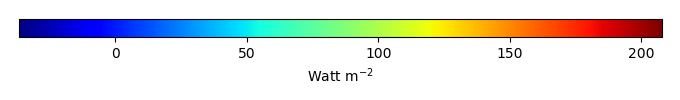MODEL MEANBIAS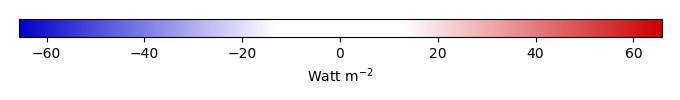BIAS SCORERMSE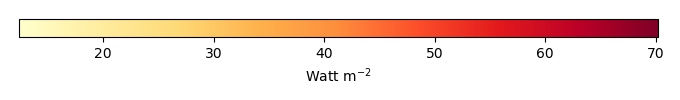RMSE SCOREBENCHMARK INTERANNUAL VARIABILITY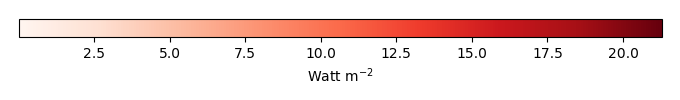MODEL INTERANNUAL VARIABILITYINTERANNUAL VARIABILITY SCOREBENCHMARK MAX MONTHMODEL MAX MONTHDIFFERENCE IN MAX MONTH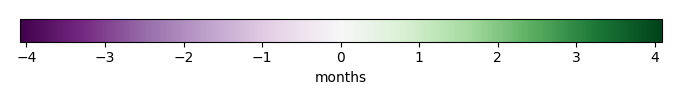SEASONAL CYCLE SCORESPATIAL TAYLOR DIAGRAMMODEL COLORS# Spatially integrated regional mean

MODEL COLORSREGIONAL MEANANNUAL CYCLEMONTHLY ANOMALYANNUAL CYCLE# All Models

BenchmarkCRUNCEPv7GSWP3v1WFDEI# Data Information

creation_date: Tue Jul 1 08:16:58 PDT 2014

source_file: This product is generated from monthly 1 degree GEWEX SRB Radiation observations